# TSA Whiteness Test VI

LabVIEW 2014 Advanced Signal Processing Toolkit Help

Edition Date: June 2014

Part Number: 372656C-01

»View Product InfoDownload Help (Windows Only)

Owning Palette: Statistical Analysis VIs

Estimates the randomness of a univariate time series and plots the correlogram. Wire data to the Xt input to determine the polymorphic instance to use or manually select the instance.

Use the pull-down menu to select an instance of this VI.

 Select an instance TSA Whiteness Test (Waveform)TSA Whiteness Test (Array)

## TSA Whiteness Test (Waveform)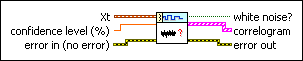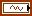Xt specifies the univariate time series.confidence level specifies the level of confidence as a percentage this VI uses to estimate the randomness of the time series.error in describes error conditions that occur before this node runs. This input provides standard error in functionality.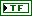white noise? returns TRUE if the univariate time series is random.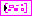correlogram returns the correlogram data including the autocorrelation of the input time series and the corresponding confidence range.error out contains error information. This output provides standard error out functionality.

## TSA Whiteness Test (Array)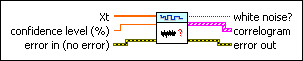Xt specifies the univariate time series.confidence level specifies the level of confidence as a percentage this VI uses to estimate the randomness of the time series.error in describes error conditions that occur before this node runs. This input provides standard error in functionality.white noise? returns TRUE if the univariate time series is random.correlogram returns the correlogram data including the autocorrelation of the input time series and the corresponding confidence range.error out contains error information. This output provides standard error out functionality.

## TSA Whiteness Test Details

This VI estimates the randomness of a univariate time series by testing the c distribution, which is implemented by calculating the statistical value e for a time series Xt with a mean of zero, as defined by the following equation: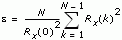where Rx(k) is the auto-correlation value of Xt, and N is the number of the input time series.

Given the confidence level a, if e<c2a/2(N–1), this statistical value passes the test of c2(N–1). You can consider this univariate time series as being a random sequence.

### Reference:

Ljung, L. 1999. System Identification Theory for the User. 2d ed. Prentice Hall.

## Example

Refer to the Series Statistical Analysis VI in the labview\examples\Time Series Analysis\TSAGettingStarted directory for an example of using the TSA Whiteness Test VI.